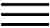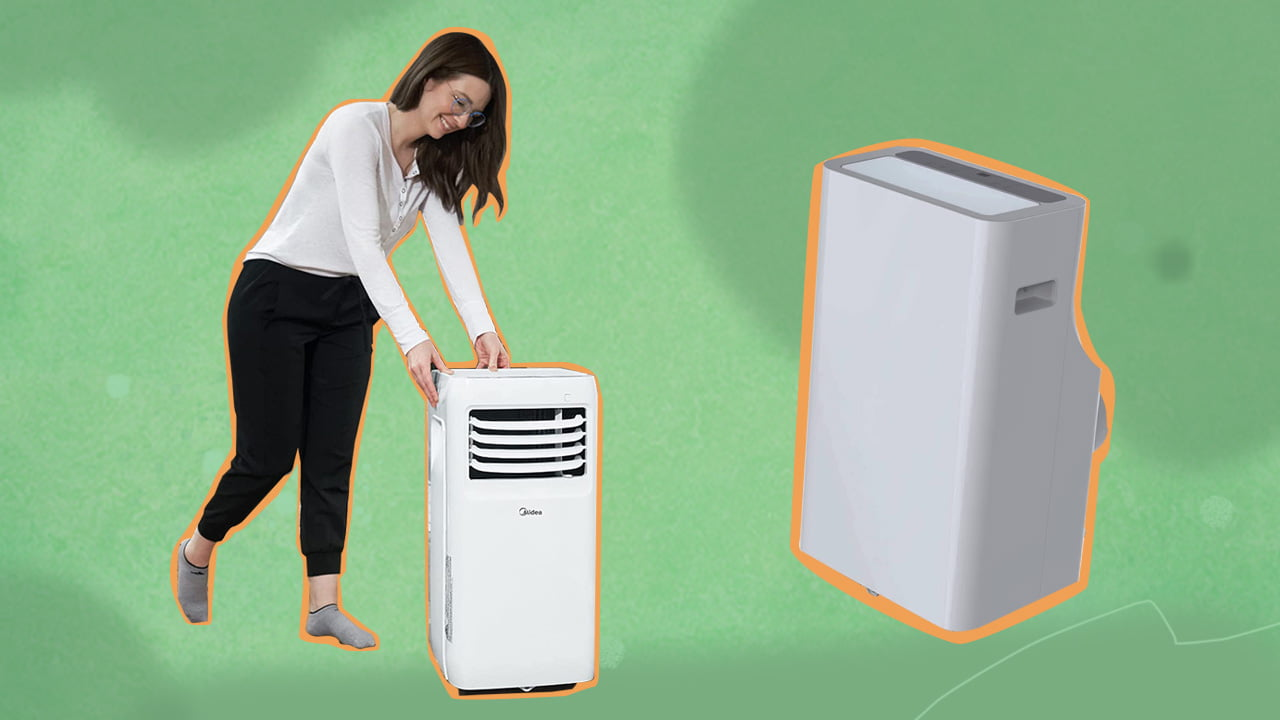X# Convert kW To BTU/h Calculator: A Simple Way To Convert Between The UnitsOne can convert from kilowatt (kW) to BTU per hour by multiplying the kW rating by 3,412. Factoring in EER, the BTU rating can be obtained by multiplying the kW rating with the EER rating and 1,000.

When looking at the cooling or heating capacity of an air conditioner, you’ll likely look at its BTU or kW rating.

The trouble is it may be difficult to gauge how powerful the model is due to one unit being more prevalent in your location. This is when you will need to convert kilowatt to BTU to determine how much heat is being removed by the AC.

So, here’s how you can convert between these power units to gauge the cooling capacity of an AC.

## Convert (kW) To BTU/hr Calculator

BTU To kW Air Conditioning Calculator

Switch

kW to BTU Air Conditioning Calculator

Switch

## Defining BTUBTUs, or British Thermal Units, is a measurement of energy that can be considered a unit of heat. One BTU is enough to raise the temperature of one pound of liquid water by a degree (Fahrenheit) at room temperature.

You may have seen BTU per hour as a unit of measurement in certain cooling units as well. This can be defined as the rate of energy transfer. The higher this value is, the more powerful the air conditioner will be. Consequently, the AC will also consume just as much power.

## Defining kW

AC specifications often come with a wattage rating, specifying how much electricity the appliance consumes. “Watt” is a basic unit of power in the SI system, a.k.a the metric system. In more physics-based terminology, it is defined as the amount of power required to move a 1 kg object with an acceleration of 1 m/s2.

Or, 1 watt = 1 Joule of work done per second

A kW is short for kilowatts, and one kilowatt has 1,000 watts. That’s the unit you see on your electricity bill.

### The Direct Equivalence Between kW And BTU

Every kilowatt is equivalent to 3,412 BTU per hour. And converting to BTU per hour from kW is a simple matter of multiplying the kW by 3,412.

For example, to calculate how many BTU/h there are in 2.5 kW, multiply the value by 3,412. The answer is 8,530 BTU/h.

### How Energy Efficiency Rating Affects This Conversion

The previous section was a direct BTU conversion from kilowatts. But, when purchasing a new air conditioner, you may want to factor in the Energy Efficiency Rating. This will paint you a clearer picture of how much power the unit consumes.

Much like the previous conversion method, this method involves simple multiplication. You can know the BTUs of an AC by multiplying the kW rating with the EER rating and then multiplying the number by 1,000. Here’s the formula presented in a simplified manner:

BTU/h = kW x EER x 1,000

For example, if the AC has a kW rating of 3 and an EER rating of 12, the result is 36. Further multiplying it by 1,000 provides 3,600 kW as a result.

Tip

Some AC units have a SEER rating listed instead of an EER rating. In such cases, you can use the formula EER = 0.875 x SEER to convert between the two.## Summing Up

It’s simple to convert kW to BTU and can be done within seconds using the calculation methods listed above. And knowing how to do so is essential when purchasing a new air conditioner. A conversion calculator is also very handy in such situations.

The reason why there are two different units for cooling capacity is due to the different measurement systems. Both the International System of Units and United States Customary units have different units, such as kilograms for the former and pounds for the latter.

This can cause some confusion, but the conversion of units is rarely a complex affair.